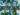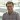# What is OpenStreetMap?

OpenStreetMap is a collaborative project to create a free editable map of the world. Map data is collected and/or edited from scratch by volunteers by using GPS data from smartphone, satellite imagery, camera etc.

The use of OpenStreetMap data has increased in recent years for both commercial and humanitarian projects. For example, a well-renowned OSM-enabled organization - Humanitarian OpenStreetMap Team (HOT) - is an international team dedicated to humanitarian action and community development through open mapping. In the events of natural hazard such as flooding, earthquake, fires etc. maps can be extremely useful for timely responses. The HOTOSM project is aimed to delegate these scenarios.

# Where does UP42 come in?

The following example shows how UP42 platform can be used to map the impact of a flooding event. This example leverages the UP42 SDK, which enables quick access and analysis of OSM and other geospatial data.

# Flood Mapping

For this example, we will look at massive flooding events in the US Midwest in 2019. The area we have chosen in Bellevue near Omaha. In order to do this we need:

1. Cloud-free satellite imagery over AOI
2. Building footprints in the same area to assess the impacted buildings

We start by importing all the required Python packages:

``````import os
from functools import partial

import geopandas as gpd
import matplotlib.pyplot as plt
import numpy as np
import pandas as pd
import pyproj
import rasterio as rio
from rasterio import features
from rasterio.plot import reshape_as_raster, show
from shapely.geometry import LineString, MultiPolygon, Point, Polygon, box
from shapely.geometry import shape as shapely_shp

import folium
import osmnx as ox
import up42``````

To get started with UP42 SDK, we will need to create a project via the browser console and get the project authentication keys. These keys need to be stored as `config.json` in the current working directory. A config file should be structured as follows:

``````{
"project_id": "abc",
"project_api_key": "xyz"
}``````

Now that we have all the prerequisites covered, we can initialize the project, run our first job and download the Sentinel-2 AOI clipped image over the area of interest.

``````# authenticate with config.json
up42.authenticate(cfg_file="config.json")

# Initialise project and workflow
project = up42.initialize_project()
workflow = project.create_workflow(name="flooding_sentinel")``````

In order to run the job, we need to provide few parameters. For this example we will use Sentinel-2 L1C MSI AOI clipped data block. A full list of data blocks available through UP42 can be found in the UP42 Marketplace.

In addition, we also need `start_date`, `end_date`, `AOI` and `geometry_operation` as input parameters. UP42 SDK provides a way to add the AOI to the workflow by supplying a valid geojson file. The chosen `AOI.geojson` for this example is located here.

This is how it looks like in code:

``````input_tasks = ['sentinelhub-s2-aoiclipped']

# Update workflow object with our desired data block as input_task(s)

# Construct input parameters
input_parameters = workflow.construct_parameters(geometry=aoi,
geometry_operation="contains",
start_date="2019-03-21",
end_date="2019-03-21",
limit=1)

# Run the actual job
job = workflow.run_job(input_parameters=input_parameters, track_status=True)``````

Once the job finishes (~2 mins) running, we can download the data as well as quicklooks with:

``````# define download directory
sentinel_dir = "./results/sentinel/"

Now that the Sentinel-2 data is downloaded, we can extract the flooded area by computing Modified Normalized Difference Water Index (MNDWI)

## Modified Normalized Difference Water Index (MNDWI)

MNDWI is computed using green and SWIR (Short-Wave Infrared) bands for enhancement of open water features. MNDWI does a great job at diminishing the false water signal due to built-up areas compared to other similar indexes.

For Sentinel data, band-3 and band-11 correspond to Green and SWIR respectively. The MNDWI is calculated with following formula:

MNDWI = (B03 - B11) / (B03 + B11)

Before we dive right into the logic of extracting flooding extent, we need to define two helper functions for computation.

``````def normalize(array):
"""Normalizes numpy arrays into scale 0.0 - 1.0"""
array_min, array_max = array.min(), array.max()
return ((array - array_min)/(array_max - array_min))

def calc_mndwi(green_band, swir_band):
"""Compute Modified Normalized Different Water Index

MNDWI = (Green - SWIR) / (Green + SWIR)
"""
mndwi = (green_band - swir_band) / (green_band + swir_band)
return mndwi.astype(np.float32)``````

Next, we compute MNDWI

• Extract pixels that indicate water
• Vectorize water pixels
``````raster_path = "path/to/sentinel/tif"
ql_path = "path/to/sentinel/quicklook/jpg"

with rio.open(raster_path) as src:

# Normalize band arrays
green_n = normalize(green)
swir_n = normalize(swir)

# get bounds,crs and transform
src_bounds = src.bounds
src_crs = src.crs
src_transform = src.transform

# Compute MNDWI
mndwi = calc_mndwi(green_band=green_n, swir_band=swir_n)``````

Our MNDWI can be quickly plotted with:

``````plt.figure(figsize=(10, 7))
plt.imshow(mndwi, cmap='terrain_r')
# Add colorbar to show the index
plt.colorbar();``````MNDWI Values Plot

Now that we have computed MNDWI, the next step is to isolate boundaries of the flooded area from the whole image.

This means,

• Applying a deterministic decision boundary / threshold to MNDWI values;
• Translating the threshold values into boolean value mask (1/0);
• Converting the raster into a vector boundary (multipolygon).

According to this paper:

For the second variant of the NDWI, another threshold can also be found in that avoids creating false alarms in urban areas:

• < 0.3 - Non-water
• >= 0.3 - Water

This means that all the values that are >= 0.3 will be mapped to value true and < 0.3 to false respectively.

``````# Create numpy mask
mndwi_msk = (mndwi >= 0.3)

# Convert pixels to shapely geometry
mypoly=[]
for vec, val in features.shapes(source=mndwi_msk.astype(np.float32), transform=src_transform):
mypoly.append({'geom': shapely_shp(vec), 'value': val})``````

For ease of performing vector based operations and vizualizations, we will convert the geometry into a `GeoDataFrame`.

``````# To gdf
submerged = gpd.GeoDataFrame(mypoly, crs=src_crs, geometry='geom')

# Filter by water area: 1 = water, 0 = non-water
submerged = submerged[submerged['value'] > 0]

# Create boundary

# Quick plot
submerged.plot(figsize=(15, 10));``````Flooded Area Vectorized

Now that we have vectorized boundaries of the flooded area which we will use to intersect with building footprint polygons from OSM. It is better to collapse all individual polygons into a single Multipolygon geometry.

Also, note the x and y axis values! (HINT: crs)

## OSM data retrieval

In this section, we will now retrieve building footprints from OpenStreetMap. At UP42, we recently developed an OpenStreetMap Data Block which is available on our marketplace.

As hinted before, the coordinate system of Sentinel-2 data is in `EPSG:3857` and the OSM query is done in `EPSG:4326`. Therefore, we'll need to perform some coordinate transformation here for both the OSM data retrieval as well as plotting the results with Folium. Let's define the transform.

``````# Transform crs
crs_project = partial(
pyproj.transform,
pyproj.Proj(init='epsg:3857'), # source projection
pyproj.Proj(init='epsg:4326') # destination projection
)

# Read dataset bounds and transform to wgs84
osm_poly = transform(crs_project, box(*src_bounds))``````

In the next part, we will create a new workflow within the scope of the same project that we initialized and authenticated earlier in this example.

``````workflow_osm = project.create_workflow(name="flooding_osm")

# Input task name for the OSM

# Update workflow object with our desired data block as input_task(s)

# Construct input parameters
input_parameters = workflow_osm.construct_parameters(geometry=aoi,
geometry_operation="bbox",
start_date="2019-03-21",
end_date="2021-03-21")``````

The job is almost ready to be run. If you notice carefully, the `end_date` parameter is in the future. The block converts the future date to today's date.

We have constructed the `input_parameters` but we haven't yet mentioned that we want to retrieve `building_footprints` from OSM. Currently, the block offers retrieval of four feature types by providing keywords listed in the table below.

OSM feature       Input Parameter (`osm_tags`)
Roads       `street_network`
Water bodies       `water_bodies`
Building footprints       `building_footprints`
Land use       `land_use`

Here, the variable `input_parameters` are nothing other than a native python `dict`. We can add `osm_tag = ["building_footprints"]` to this dictionary. The key `osm_tag` can be a list of all above tags as well.

``````# add osm_tags to input_parameters
input_parameters['openstreetmap:1']["osm_tags"] = ["building_footprints"]

# Run the job
job_osm = workflow_osm.run_job(input_parameters=input_parameters, track_status=True)

osm_dir = "./results/osm/"

Once the job finishes running, we can download the `geojson` results and read it to `GeoDataFrame` with correct projection

``````gdf = gpd.read_file("./results/osm/building_footprints.geojson")

# Reproject to EPSG:3857
gdf_proj = gdf.to_crs(src_crs)

# Plot
gdf_proj.plot(figsize=(15, 10))``````Building Footprints Retrieved with OSM Block

## Extract Flood Impacted buildings

Until this point, we set the stage for the actual task. Basically, the building footprints that intersects the Multipolygon (one we created from MNDWI) are affected buildings and those that are not are non-affected buildings!

``````effected = gdf_proj[gdf_proj['geometry'].intersects(boundary)]
not_effected = gdf_proj[~gdf_proj['geometry'].intersects(boundary)]

# Change coordinate system for plotting because folium wants 4326
effected.to_crs(crs='EPSG:4326', inplace=True)
not_effected.to_crs(crs='EPSG:4326', inplace=True)``````

## Plotting

Now, we have everything required to see the impact on a map. We will use the Folium plotting library for this. But first, we will define some parameters for better visualization.

``````# bbox centroid serves as the center point for the folium map
bbox_centroid = list((osm_poly.centroid).coords[:])
bbox_centroid = [bbox_centroid[-1], bbox_centroid]

# Extracts bounds for image overlay
lon_min, lat_min, lon_max, lat_max = osm_poly.bounds

style1 = {'fillColor': '#228B22', 'color': 'red'}
style2 = {'fillColor': '#00FFFFFF', 'color': '#00FFFFFF'}``````

Finally we can plot our results in an interactive map!

``````# init folium map
m = folium.Map(bbox_centroid, zoom_start=15)

folium.raster_layers.ImageOverlay(image=ql_path, bounds=[[lat_min, lon_min], [lat_max, lon_max]], opacity=0.8).add_to(m);``````Final map - impacted buildings in red

As we can see the buildings impacted by flooding are in red and those that are not impacted are in blue. This is just one of many use cases where OSM data and UP42 provides great value. However, It should be noted that success of the analysis depends on the availability of the data in OSM! If the data is not available in OSM, you can also try one of our Building Detection partner blocks from Aventior to extract building footprints! Or start contributing to OSM and give the community back!

References:
1: Space4Water Portal - MDNWIPrayag Thakkar

Data Science Engineer at UP42

### Start building with UP42

Ready to get started? Create an account in seconds and we'll even give you 10,000 credits to get your first insights.